Fit Analyses

# Generalized Linear Models

Generalized linear models assume that the response yi has a distribution from the exponential family (normal, inverse Gaussian, gamma, Poisson, binomial) and a function can be used to link the expected response mean and a linear function of the X effects. In SAS/INSIGHT software, a generalized linear model is written as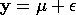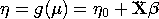where y is the n×1 vector of responses,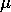is the n×1 expected response means, andis the n×1 vector of unknown errors.

The monotone function g links the response meanwith a linear predictor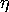from the effects, and it is called the link function. The n×1 vector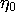is the offset, X is the n×p design matrix, andis the p×1 vector of unknown parameters. The design matrix is generated the same way as for linear models.

You specify the response distribution, the link function, and the offset variable in the fit method options dialog.